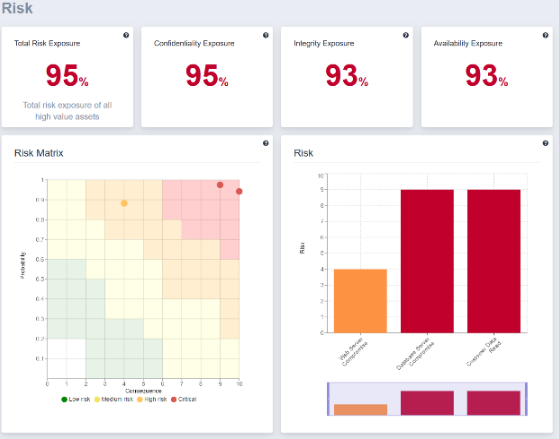# Risk overview

The Total Risk value denotes the sum of the total expected risk of all assets with consequences set on them. It is calculated by taking the user defined consequence of all attack steps multiplied by its respective probability of an attacker reaching that attack step. The gauge shows the current risk in proportion to the sum of all user defined consequences.

Confidentiality Risk denotes the total percentage of expected loss of confidentiality on all assets with consequences set on them. The value is calculated by summarizing the probability of success of an attacker reaching attack steps that affects confidentiality.

Integrity Risk denotes the total percentage of expected loss of integrity on all assets with consequences set on them. The value is calculated by summarizing the probability of success of an attacker reaching attack steps that affects integrity.

Availability Risk denotes the total percentage of expected loss of availability on all assets with consequences set on them. The value is calculated by summarizing the probability of success of an attacker reaching attack steps that affects availability.

The Risk Matrix is a matrix that is used during risk assessment to define the various levels of risk of attacks. On the vertical axis, we have the probability for the attack to succeed within a given number of days. On the horizontal axis, we have the consequence estimates between 0 and 10.